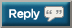# Thread: 2017 Push-up Challenge - try for 40,000 this time

1. 30,631 + 260 = 30,8912. 30,891 + 250 = 31,1413. 31,141 + 116 = 31,2574. 31,257 + 410 = 31,6675. I do 60 push-ups each week, so from the beginning of the year, I've done 1,140 push-ups.

31,667
+1,140
='s 32,8076.7. 33,027 + 100 = 33,1278. 33,127 + 54 = 331819. 33,181+ 105 = 33,28610.11. I have done 1420 so far this year, so that is:

33886 + 1420 = 35,30612. I have done 1420 so far this year, so that is:

33886 + 1420 = 35,306
I am up to around 6,500 for the year...this thread keeps me accountable

35,306 + 280 = 35,58613. I am up to around 6,500 for the year...this thread keeps me accountable

35,306 + 280 = 35,586
Last couple of days: 400 + 35,586 = 35,98614. Last couple of days: 400 + 35,586 = 35,986
35,986 + 200 = 36,18615. + 60 = 36,246

There's some butt kickin going on in here...good job guys!16. + 60 = 36,246

There's some butt kickin going on in here...good job guys!
36,246 +50 = 36,296

Love me some pushups!17. 36,246 +50 = 36,296

Love me some pushups!
36,296 + 580 = 36,87618. 36,876 + 250 = 37,12619. Haven't updated in a while so got a big old bump for this:

37,126 + 1,255 = 38,381

Almost there guys!20. Haven't updated in a while so got a big old bump for this:

37,126 + 1,255 = 38,381

Almost there guys!
38381 + 200 = 3858121. 38,581 + 200 = 38,78122. 38,781 + 270 = 39,05123. 39,051 + 60 (AKA 2,000 'NoCarbs/NoSugar' pushups) = 39,11124. 39,051 + 60 (AKA 2,000 'NoCarbs/NoSugar' pushups) = 39,111
+320=39,43125.26.27.28. 40,267 + 260 = 40,52729. new daily pushup PR for me!

40,527 + 320 = 40,84730. new daily pushup PR for me!

40,527 + 320 = 40,847
40,847 + 250 = 41,097#### Posting Permissions

• You may not post new threads
• You may not post replies
• You may not post attachments
• You may not edit your posts